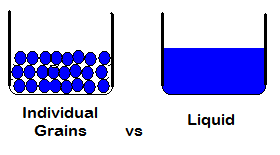# Quantity Measurements. Choosing the Correct Procedure.

## Quantity Measurement is Important

Quantity is a pretty easy concept to grasp.  But, it isn’t always easy to measure.  Quantity is basically an amount of something.  But different substances are easier to measure than others.  For example, if you have a cup full of marbles, you may be able to pour them out and count them individually.  What if you have a cup full of rice?  How many grains of rice do you have?  There are ways of measuring quantity other than just counting.  The two most common ways are by using a volume measurement, or a weight measurement.

If you go to the grocery store most of the packaged food is going to be measured by either weight, or volume.  Some of the more common weight measurements on packages for sale in the US would be ounces and pounds.  Some of the common volume measurements would be cups, pint, quarts and gallons.  So, what type of measurement do you use when trying to determine quantity?  It really depends on the physical attributes of the substance you’re measuring.

## Quantity, How Much Is In The Jar?

When you are measuring a non-liquid substance, you will normally use weight at a measurement tool to determine quantity.  The reason you don’t use volume with a group of solid substances is because you can’t account for the tiny space in between the individual units.  For example, if you fill one cup with water, and one cup with ice cubes, you’ll notice that the ice cubes have space in between them, while the water completely fills the entire container.When using weight as a measurement for quantity, you will typically weigh a sample of the substance individually, then measure the entire group.  If you take the weight of the entire group and divide it by the weight of the individual sample piece, you can approximate how many total pieces there are.

Typically, when measuring the quantity of a liquid, you’re going to use volume.  Because a liquid will completely fill the container, you can fairly accurately determine the quantity of the liquid by the volume filled in the container.

For online calculators for volume or weight go to CalcuNATION.com.

Online Science Calculators

Online Calculators for Volume Measurements

Online Calculators for Volume and Area Measurements

CalcuNATION is a website featuring online calculators and educational resources for mathematics.  Other Mathematical Blogs ( CalcuNATION on EduBlogs and CalcuNATION on Blogger)

Quantity Measurements. Choosing the Correct Procedure.
Scroll to top